## Filters

Sort by :
Clear All
Q
Engineering
149 Views   |

The potential (in volts) of a charge distribution is given bydoes not depend on x and y. If this potential is generated by a constant charge per unit volume ρ0 (in units of) which is spread over a certain region, then choose the correct statement.

• Option 1)• Option 2)• Option 3)• Option 4)As we learnt in Electric Potential due to Continious charge distribution - -                           Now The source is an infinite non conducting thick plate of thickness  2m Option 1) Correct Option Option 2) Incorrect Option Option 3) Incorrect Option Option 4) Incorrect Option
Engineering
546 Views   |

Three capacitors each of 4 µF are to be connected in such a way that the effective capacitance is 6 µF. This can be done by connecting them :

• Option 1)

all in series

• Option 2)

two in series and one in parallel

• Option 3)

all in parallel

• Option 4)

two in parallel and one in series

As we learnt in

Series Grouping -

- wherein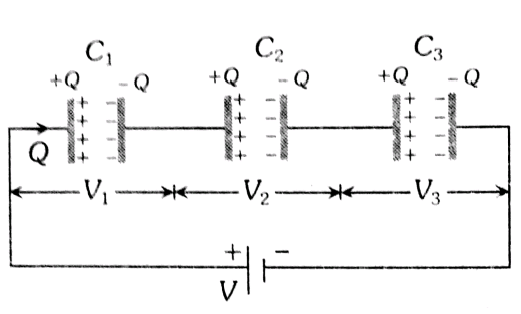Parallel Grouping -

- wherein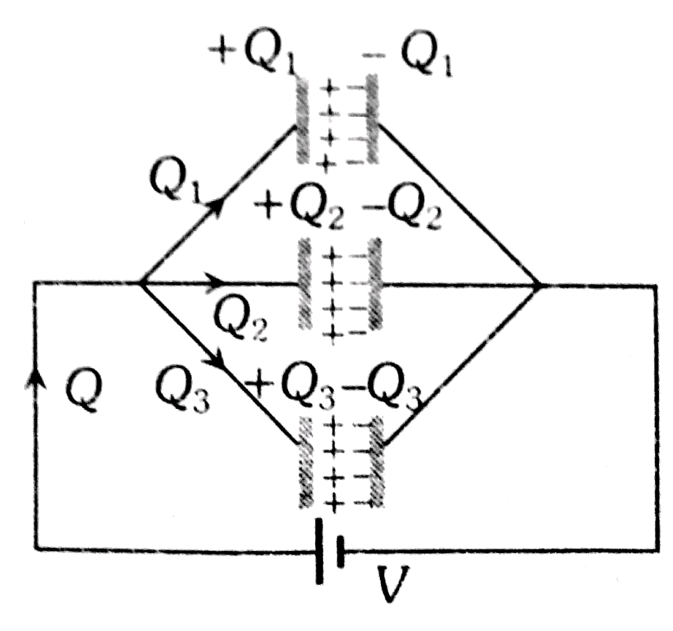Option 1)

all in series

Incorrect Option

Option 2)

two in series and one in parallel

Correct Option

Option 3)

all in parallel

Incorrect Option

Option 4)

two in parallel and one in series

Incorrect Option

View More
Engineering
556 Views   |

Within a spherical charge distribution of charge density  ρ(r), N equipotential surfaces of potential V0, V+ V, V0 + 2V, .......... V0 + NV  (V>0), are drawn and have increasing radii r0, r1, r2,..........rN, respectively.  If the difference in the radii of the surfaces is constant for all values of V0 and V then :

• Option 1)• Option 2)• Option 3)• Option 4)As we learnt in

Relation between field and potential -

- wherein

If P at the surface r = R -

-

We know

Here  and  are same for any pair of surfaces.

E= constant

Now, electric field inside the spherical charge distribution

E would be constant of

Option 1)Incorrect option

Option 2)Incorrect option

Option 3)Correct option

Option 4)Incorrect option

View More
Engineering
878 Views   |

Figure shows a network of capacitors where the numbers indicates capacitances in micro Farad.  The value of capacitance C if the equivalent capacitance between point A and B is to be 1 µF is :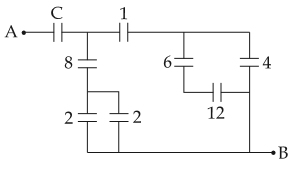• Option 1)• Option 2)• Option 3)• Option 4)As we learnt in

Series Grouping -

- whereinParallel Grouping -

- whereinOption 1)Incorrect

Option 2)Correct

Option 3)Incorrect

Option 4)Incorrect

View More
Engineering
128 Views   |

The region between two concentric spheres of radii ‘a’ and ‘b’, respectively (see figure), has volume charge densitywhere A is a constant and r is the distance from the centre.  At the centre of the spheres is a point charge Q.  The value of A such that the electric field in the region between the spheres will be constant, is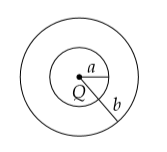• Option 1)• Option 2)• Option 3)• Option 4)As we learnt in

Gauss's Law -

Total flux linked with a closed surface called Gaussian surface.

Formula:

- wherein

No need to be a real physical surface.

Qenc - charge enclosed by closed surface.

Now putting the value of q in equation (i)

E will be constant if it is independent of r

Option 1)Correct

Option 2)Incorrect

Option 3)Incorrect

Option 4)Incorrect

View More
Engineering
1419 Views   |

A combination of capacitors is set up as shown in the figure.  The magnitude of the electric field, due to a point charge Q (having a charge equal to the sum of the charges on the 4 µF and 9 µF capacitors), at a point distant 30 m from it, would equal :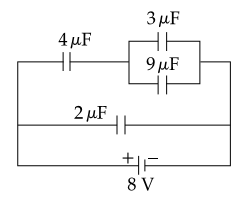• Option 1)

240 N/C

• Option 2)

360 N/C

• Option 3)

420 N/C

• Option 4)

480 N/C

As we learnt in

Series Grouping -

- whereinParallel Grouping -

- whereinElectric Field Intensity -

- wherein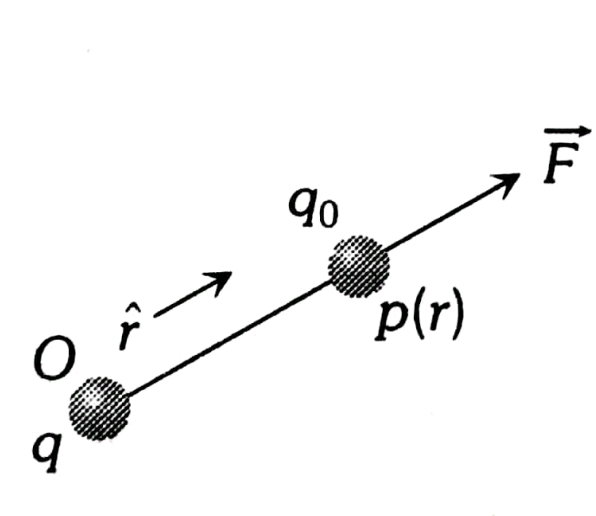are in parallel combinations so their equivalent capacitance is

Now  are in series sso their equivalent capacitance

Charge on  =

Charge on  are same

as they are in series

Charge on

Now  required charge on Q = charge on       +     charge on

Option 1)

240 N/C

Incorrect

Option 2)

360 N/C

Incorrect

Option 3)

420 N/C

Correct

Option 4)

480 N/C

Incorrect

View More
Exams
Articles
Questions